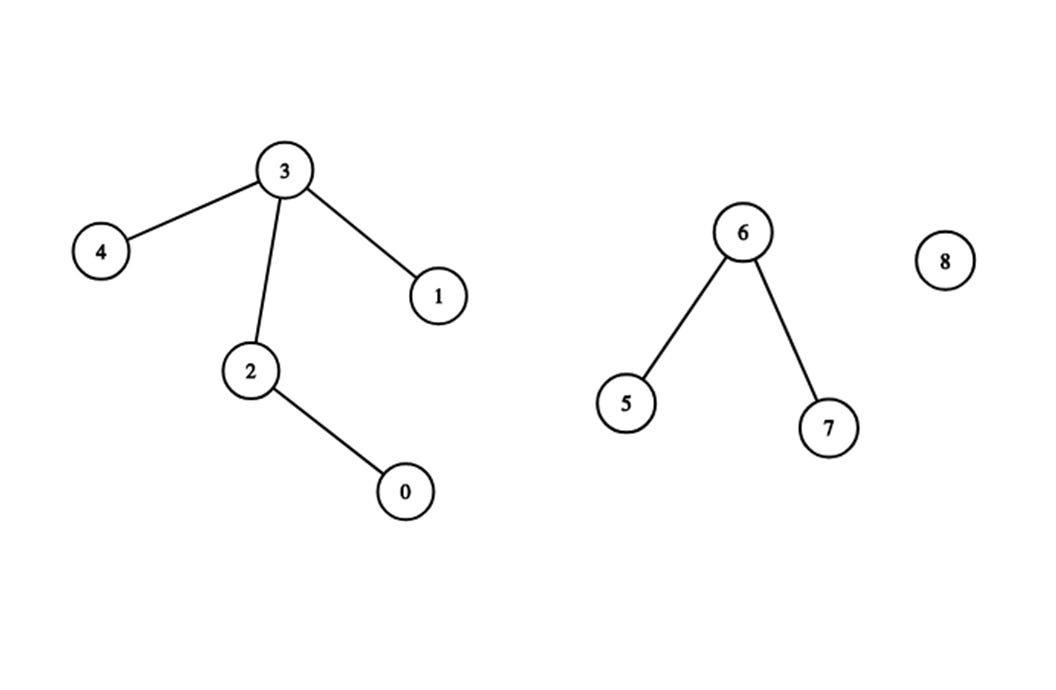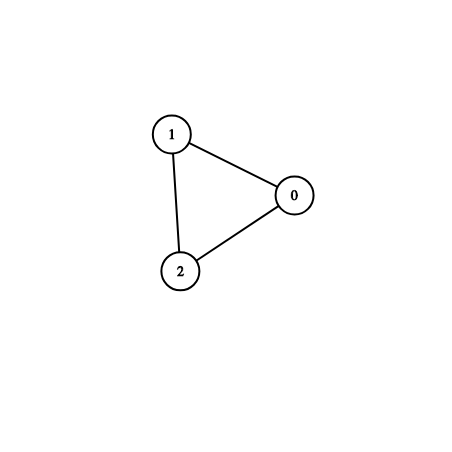# Disjoint-set union in C++ and Swift

Have you ever wondered, when you drive by your car, how your navigation system corrects the optimal route, from your current position to your destination, on changes based on the input data such as traffic jams and missed turns?

This is one of the real-time practical application of the Minimum Spanning Tree (MST) problem, in which an algorithm like Kruskal’s Algorithm tries to solve. In this article, we will learn the main data structure that’s used in the Kruskal Algorithm in order to solve the MST problem in an efficient way.

In this article, we will be quickly going through:

### Introduction

Imagine having a graph, like what’s in the following image. You are asked to make two queries really fast which are:
1. unionSets i.e. merge two sets together.
2. isSameSet i.e. find wither the two nodes are in the same set or not.Image from https://csacademy.com/app/graph_editor/
The Disjoint-set data structure allows us to very quickly determine if two items are in the same set (or equivalently determine if two vertices are in the same connected component), and also to very quickly unite two sets (or equivalently combine two connected components into one connected component).

A union-find algorithm is an algorithm that performs two useful operations on such a data structure:
Find: Determine which subset a particular element is in. This can be used for determining if two elements are in the same subset.
Union: Join two subsets into a single subset.

Union-Find Algorithm can be used to check whether an undirected graph contains a cycle or not. This method assumes that the graph doesn’t contain any self-loops. And we can keep track of the subsets in a 1D array.

### How it works

In the following graph, let’s find out buy this union-find algorithm wither this is graph has a cycle or not.Image from https://csacademy.com/app/graph_editor/

For each edge in this graph make subsets using both the vertices of the edge. If both the vertices are in the same subset, a cycle is found.

Then make a parent array and memset it to -1 (i.e. make -1 as the initial value for all of the array). Then process all of these edges one by one.

` 0  1  2 --> the nodes.-1 -1 -1 --> the parent array.`Image from http://visualgo.net
Edge 0–1: Find the subsets in which vertices 0 and 1 are. Since they are in different subsets, we take the union of them. For taking the union, either make node 0 as the parent of node 1 or vice-versa.
` 0  1  2 --> the nodes. 1 -1 -1 --> node number 1 is the parent of node 0.`
Edge 1–2: 1 is in subset 1 and 2 is in subset 2. So, take union.
`0  1  2 --> the nodes.1  2 -1 --> node number 2 is the parent of node 1.`
Edge 0–2: 0 is in subset 2 and 2 is also in subset 2.
Thus, including this edge forms a cycle.

### Implementation

#### In C++

Let’s start with creating a new class and call it DSU. If you are familiar with C++, your class will have a .h and a .cpp files.

We will create the parent array and the sz array dynamically, also we will need a private method to get the parent of the current node, and finally, in the private members' section, we will need a swap method.

In the public members’ section, we need a constructor that will take the size of our graph, unionSets method to merge two sets together, isSameSet method to find out wither the two nodes are in the same set or not, and finally, of course, we need a destructor.

While implementing those methods, start with the find_parent().
The idea here is to save the parent of the nodes in the parent array, therefore when you need this information it will give you the info in an amortized O(1) complexity rather than O(n).

In same_set() method we will just compare the parent of both nodes. If they match then they are in the same set. Finally, in union_sets() method, you will find the parent for both nodes then union them. If they aren’t in the same set.

The constructor will fill the parent array with the number of nodes starting from 0 to n.

#### In Swift

Create a new Swift Playground, then create a class call it DSU. In this step, I’ll be converting the C++ code to Swift. I quickly came up with something very similar.

Note, I needed to change properties in place so I used the inout keyword for the function’s parameters.

All parameters passed into a Swift function are constants, so you can’t change them. If you want, you can pass in one or more parameters as `inout`, which means they can be changed inside your function, and those changes reflect in the original value outside the function.

All of the code in this article is on my GitHub.

### Conclusion

This data structure is used by the Boost Graph Library to implement its Incremental Connected Components functionality. It is also a key component in implementing Kruskal’s algorithm to find the minimum spanning tree of a graph.

I had fun playing with it and solving some MST problems in C++, and I really learned a lot by just implementing this data structure. Therefore, I wanted to try implementing it in Swift.

Thanks for reading and I hope you had fun reading this article. If you have any thoughts please contact me over twitter.

### Resources

You can read more about DSU from: